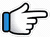# Jab Koi Baat Bigad Jaye – Keyboard Notes## Jab Koi Baat Bigad Jaye Keyboard Notes

For Jab Koi Baat Bigad Jaye Notes as Western or Indian Notations, Click –

Western – CDE FGAB

Indian – srg mpdn

Hindi – सारेग मपधनि

## Keyboard Notes for Jab Koi Baat Bigad Jaye – Indian Notation (srg mpdn)

CDE FGAB

सारेग मपधनि

Music:
D~ pD~~    D~ pD~~
D~ pD~~    R*~ nd~

d~ Dd~~    d~ Dd~~
d~ DM~~   n~ dD~~

dDM~  DMg~

gg      g~     D~~
Jab    koi    baat
Or
g~      gg     D~~

DM         g~ D

M      g       MD
jab    koi    mushkil

M        g~ R~
Or
M       gMg~ R

Rg       M~ D    M~ g      R *n~~
Tum   dena     saath      mera
Or
Rg     M~ D     M~ g    RgR *n

*n      R~ gg~~
O       humnawaaz
Or
*n      R~ gRg

Repeat:
gg     g~    D~~   DM      g~ D

M      g      MD           M      g~ R~

Rg       M~ D    M~ g      R *n~~
Tum   dena     saath      mera

*n      R~ gRg
O       humnawaaz

g         g~ g~    D~
Naa    koi        hai

D  M~ g~     D
na koi         tha

M~ Dg M           DM  g          gR
zindagi_ mein   tumhaare    siva
Or
M~ Mg M          DM  g          gR
D~ Mg M           DM  g         MgR

Rg      MD     Mg        gRgR *n
Tum  dena    saath    mera

*n    R~  gRgMgR
O     hum~nawaa~~z

Repeat tum dena but notes are different for o humnawaaz

Rg       MD      Mg       RgR *n
Tum   dena    saath   mera

*n     d~  DMDMg
O      hum~nawaa~~z

Music:
D~ pD~~    D~ pD~~
D~ pD~~    R*~ nd~

d~ Dd~~    d~ Dd~~
d~ DM~~   n~ dD~~

dDM~  DMg~

nN n~ nN          n      nN    n
Ho_ chaandni  jab   tak   raat
Or
n~    n~ nN         n     nN~    n

n~ N    n     ng*    R*~n  d
deta   hai   har     koi    saath

dR*       R*R*
Tum     magar

R*R*~ R*r*      r*R* R* n
andheron         me_in~

n      nMMn      d~ D      D
na   chhodna   mera    haath
Or
n~    M~ Mn    d~ D     D

Repeat:
Ho chandni jab..

dDM~  DMg~

gg      g~     D~~   DM      g~ D

M      g       MD         M      g~ R~

Rg      M~ D     M~ g     R *n~~
Tum  dena      saath    mera

*n     R~ gRg
O      humnawaaz

g         g~ g~    D~    D  M~ g~     D
Naa    koi        hai   na koi         tha

M~ Dg M             DM  g           gR
zindagi_ mein     tumhaare    siva

Rg       MD       Mg       RgR *n
Tum    dena    saath    mera

*n     d~  DMg
O      hum~nawaa~~z

nN      nn        N     n      nNn
Wafa_daari   ki     vo    rasmein

n~ N   nn          ng*   R*~    ndd
nibha_yenge   hum_ tum   kasmein

d~     R*R*    R*~ R*
Ek     bhi       saans

R*~ R*R*r*
zindagi    ki

r*R*R*n   n    nMMn   dD     D
jab_tak     ho  apne       bas  mein

Repeat:
Wafa_daari   ki     vo    rasmein

gg      g~     D~~   DM      g~ D

M      g       MD         M      g~ R~

Rg      M~ D     M~ g     R *n~~
Tum  dena      saath    mera

*n     R~ gRg
O      humnawaaz

g         g~ g~    D~    D  M~ g~     D
Naa    koi        hai   na koi         tha

M~ Dg M             DM  g           gR
zindagi_ mein     tumhaare    siva

Rg       MD       Mg       RgR *n
Tum    dena    saath    mera

*n     d~  DMg
O      hum~nawaa~~z

Song List

Bhajan List

New Song List